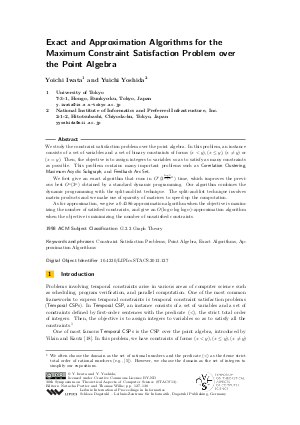Document# Exact and Approximation Algorithms for the Maximum Constraint Satisfaction Problem over the Point Algebra

### Authors Yoichi Iwata, Yuichi Yoshida## File

LIPIcs.STACS.2013.127.pdf
• Filesize: 0.58 MB
• 12 pages

## Cite As

Yoichi Iwata and Yuichi Yoshida. Exact and Approximation Algorithms for the Maximum Constraint Satisfaction Problem over the Point Algebra. In 30th International Symposium on Theoretical Aspects of Computer Science (STACS 2013). Leibniz International Proceedings in Informatics (LIPIcs), Volume 20, pp. 127-138, Schloss Dagstuhl - Leibniz-Zentrum für Informatik (2013)
https://doi.org/10.4230/LIPIcs.STACS.2013.127

## Abstract

We study the constraint satisfaction problem over the point algebra. In this problem, an instance consists of a set of variables and a set of binary constraints of forms (x < y), (x <= y), (x \neq y) or (x = y). Then, the objective is to assign integers to variables so as to satisfy as many constraints as possible.This problem contains many important problems such as Correlation Clustering, Maximum Acyclic Subgraph, and Feedback Arc Set. We first give an exact algorithm that runs in O^*(3^{\frac{log 5}{log 6}n}) time, which improves the previous best O^*(3^n) obtained by a standard dynamic programming. Our algorithm combines the dynamic programming with the split-and-list technique. The split-and-list technique involves matrix products and we make use of sparsity of matrices to speed up the computation. As for approximation, we give a 0.4586-approximation algorithm when the objective is maximizing the number of satisfied constraints, and give an O(log n log log n)-approximation algorithm when the objective is minimizing the number of unsatisfied constraints.
##### Keywords
• Constraint Satisfaction Problems
• Point Algebra
• Exact Algorithms
• Approximation Algorithms

## Metrics

• Access Statistics
• Total Accesses (updated on a weekly basis)
0